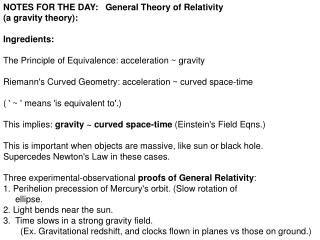DownloadDownload PresentationNOTES FOR THE DAY: General Theory of Relativity (a gravity theory): Ingredients:

NOTES FOR THE DAY: General Theory of Relativity (a gravity theory): Ingredients:

Download PresentationNOTES FOR THE DAY: General Theory of Relativity (a gravity theory): Ingredients:

- - - - - - - - - - - - - - - - - - - - - - - - - - - E N D - - - - - - - - - - - - - - - - - - - - - - - - - - -
Presentation Transcript

1. NOTES FOR THE DAY: General Theory of Relativity • (a gravity theory): • Ingredients: • The Principle of Equivalence: acceleration ~ gravity • Riemann's Curved Geometry: acceleration ~ curved space-time • ( ' ~ ' means 'is equivalent to'.) • This implies: gravity ~ curved space-time (Einstein's Field Eqns.) • This is important when objects are massive, like sun or black hole. • Supercedes Newton's Law in these cases. • Three experimental-observational proofs of General Relativity: • 1. Perihelion precession of Mercury's orbit. (Slow rotation of • ellipse. • 2. Light bends near the sun. • Time slows in a strong gravity field. • (Ex. Gravitational redshift, and clocks flown in planes vs those on ground.)

2. The General Theory of Relativity(GTR) is a new theory of gravity, beyond Newton’s, & based on two ingredients: 1. The Principle of Equivalence: acceleration ~ gravity Conceived by Ernst Mach 1838-1902 ~ means ‘is equivalent to’.

3. Einstein can’t tell whether he is on the earth or in space, accelerating at 1 g.

4. George Riemann 1826-1866 2. Riemann's Curved Geometry was another ingredient, implying acceleration ~ curved space-time

5. The essential idea of Einstein’s GTR acceleration ~ gravity and acceleration ~ curved space-time imply gravity ~ curved space-time (Einstein's Field Eqns.) This is important when objects are massive, like sun or black hole. Supercedes Newton's Law in these cases.

6. Black hole—HELP! We need Einstein!

7. Three experimental-observational proofs of General Relativity: • Perihelion precession of Mercury's orbit • (slow rotation of elliptical orbit • 16 degrees/10,000 yrs).

8. Three experimental-observational proofs of General Relativity: 2. Light bends near the sun.

9. Arthur Eddington went to South Africa during a total eclipse of the sun To test the bending of light by the sun’s gravity. He was the first to suggest this. It was later confirmed by another observation.

10. Three experimental-observational proofs of General Relativity: 3. Time slows in gravity

11. The frequency of light (time-dependent) is decreased by a massive star (toward the red end of the spectrum). This is called a gravitational red shift.